# GMAT Math : DSQ: Calculating the equation of a perpendicular line

## Example Questions

### Example Question #1 : Dsq: Calculating The Equation Of A Perpendicular Line

Line AB is perpindicular to Line BC. Find the equation for Line AB.

1. Point B (the intersection of these two lines) is  (2,5).

2. Line BC is parallel to the line y=2x.

Statement 1 alone is sufficient, but statement 2 alone is not sufficient to answer the question.

Both statements taken together are sufficient to answer the question, but neither statement alone is sufficient.

Statements 1 and 2 together are not sufficient.

Statement 2 alone is sufficient, but statement 1 alone is not sufficient to answer the question.

Each statement alone is sufficient.

Both statements taken together are sufficient to answer the question, but neither statement alone is sufficient.

Explanation:

To find the equation of any line, we need 2 pieces of information, the slope of the line and any point on the line. From statement 1, we get a point on Line AB. From statement 2, we get the slope of Line BC. Since we know that AB is perpindicular to BC, we can derive the slope of AB from the slope of BC. Therefore to find the equation of the line, we need the information from both statements.

### Example Question #2 : Dsq: Calculating The Equation Of A Perpendicular Line

Given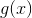, find the equation of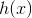, a line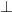to.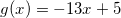I)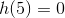.

II) The-intercept ofis at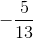.

Statement I is sufficient to answer the question, but statement II is not sufficient to answer the question.

Either statement is sufficient to answer the question.

Statement II is sufficient to answer the question, but statement I is not sufficient to answer the question.

Both statements are needed to answer the question.

Either statement is sufficient to answer the question.

Explanation:

To find the equation of a perpendicular line you need the slope of the line and a point on the line. We can find the slope by knowing g(x).

I) Gives us a point on h(x).

II) Gives us the y-intercept of h(x).

Either of these will be sufficient to find the rest of our equation.

### Example Question #3 : Dsq: Calculating The Equation Of A Perpendicular Line

Calculate the equation of a line perpendicular to line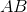.

1. The equation for lineis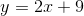.
2. Linegoes through point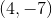.

Both statements taken together are sufficient to answer the question, but neither statement alone is sufficient.

Each statement alone is sufficient to answer the question.

Statements 1 and 2 are not sufficient, and additional data is needed to answer the question.

Statement 1 alone is sufficient, but statement 2 alone is not sufficient to answer the question.

Statement 2 alone is sufficient, but statement 1 alone is not sufficient to answer the question.

Both statements taken together are sufficient to answer the question, but neither statement alone is sufficient.

Explanation:

Statement 1: We're given the equation to line AB which contains the slope. Because the line we're being asked for is perpendicular to it, we know the slope will be its inverse.

The slope of our line is thenStatement 2: We can write the equation to the perpendicular line only if we have a point that falls within that line. Luckily, we're given such a point in statement 2.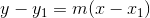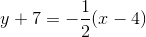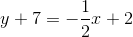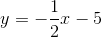Both statements taken together are sufficient to answer the question, but neither statement alone is sufficient.

### Example Question #4 : Dsq: Calculating The Equation Of A Perpendicular Line

Find the equation of the line perpendicular to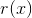.

I)has a slope of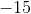.

II) The line must pass through the point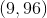.

Either statement is sufficient to answer the question.

Statement I is sufficient to answer the question, but statement II is not sufficient to answer the question.

Both statements are needed to answer the question.

Statement II is sufficient to answer the question, but statement I is not sufficient to answer the question.

Both statements are needed to answer the question.

Explanation:

Find the equation of the line perpendicular to r(x)

I) r(x) has a slope of -15

II) The line must pass through the point (9, 96)

Recall that perpendicular lines have opposite reciprocal slopes.

Use I) to find the slope of our new line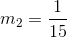Use II) along with our slope to find the y-intercept of our new line.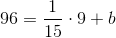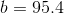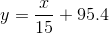Therefore both statements are needed.

### Example Question #5 : Dsq: Calculating The Equation Of A Perpendicular Line

Consider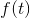: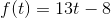Find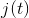, a line perpendicular to, given the following:

I)passes through the point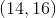.

II)passes through the point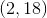.

Statement II is sufficient to answer the question, but Statement I is not sufficient to answer the question.

Both statements are needed to answer the question.

Statement I is sufficient to answer the question, but Statement II is not sufficient to answer the question.

Either statement is sufficient to answer the question.

Statement I is sufficient to answer the question, but Statement II is not sufficient to answer the question.

Explanation:

Recall that perpendicular lines have opposite, reciprocal slopes. We can find the slope offrom the question.

Statement I gives us a point on, which we can use to find the y-intercept of, and then the equation.

The slope ofmust be the opposite reciprocal of, this makes our slope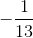.

Statement I tells us thatpasses through the point, so we can use slope-intercept form to find our equation: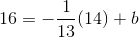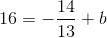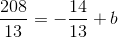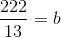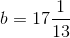So, our equation is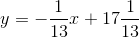Statement II gives us a point on, which does not help us in the slightest with. Therefore, only Statement I is sufficient.

### Example Question #6 : Dsq: Calculating The Equation Of A Perpendicular Line

Give the equation of a line on the coordinate plane.

Statement 1: The line shares an-intercept and its-intercept with the line of the equation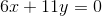.

Statement 2: The line is perpendicular to the line of the equation.

BOTH statements TOGETHER are sufficient to answer the question, but NEITHER statement ALONE is sufficient to answer the question.

EITHER statement ALONE is sufficient to answer the question.

BOTH statements TOGETHER are insufficient to answer the question.

Statement 1 ALONE is sufficient to answer the question, but Statement 2 ALONE is NOT sufficient to answer the question.

Statement 2 ALONE is sufficient to answer the question, but Statement 1 ALONE is NOT sufficient to answer the question.

BOTH statements TOGETHER are sufficient to answer the question, but NEITHER statement ALONE is sufficient to answer the question.

Explanation:

Assume Statement 1 alone. The-intercept of the line of the equation can be found by substituting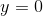and solving for: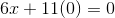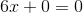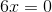The-intercept of the line is the origin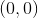; it follows that this is also the-intercept.

Therefore, Statement 1 alone yields only one point of the line, from which its equation cannot be determined.

Assume Statement 2 alone. The slope of the line of the equationcan be calculated by putting it in slope-intercept form: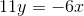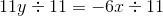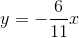The slope of this line is the coefficient of, which is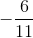. A line perpendicular to this one has as its slope the opposite of the reciprocal of, which is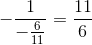.

However, there are infintely many lines with this slope, so no further information can be determined.

Now assume both statements to be true. From Statement 1, the slope of the line is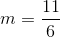, and from Statement 2, the-coordinate of the-initercept is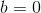. Substitute in the slope-intercept form: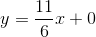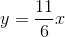### Example Question #7 : Dsq: Calculating The Equation Of A Perpendicular Line

Find the equation to a line perpendicular to line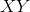.

1. The slope of lineis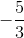.
2. Linegoes through point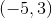.

Statement 2 alone is sufficient, but statement 1 alone is not sufficient to answer the question.

Both statements taken together are sufficient to answer the question, but neither statement alone is sufficient.

Each statement alone is sufficient to answer the question.

Statement 1 alone is sufficient, but statement 2 alone is not sufficient to answer the question.

Statements 1 and 2 are not sufficient, and additional data is needed to answer the question.

Both statements taken together are sufficient to answer the question, but neither statement alone is sufficient.

Explanation:

Statement 1: Since the line we're looking for is perpendicular to line XY, our slope will be the inverse of line XY's slope.

The slope of our line is then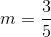. Just knowing the slope however, is not sufficient information to answer the question.

Statement 2: We're provided with a point which will allow us the write the equation.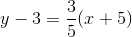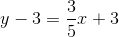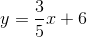Tired of practice problems?

Try live online GMAT prep today.